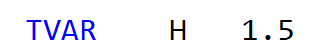# [Bug Report] Axis Variable doesn't work at all?

First of all, here is the Teddy .dat file link: three span box girder bridge.dat (4.8 KB)

Basiclly it’s a 3-span concrete single box with two cells girder bridge.

The cross section has 26 independent variables, like ‘H’ - box total height, ‘Wt’ - top slab width, ‘Tt’ - top slab thickness, etc

The cross section also has 38 derived variables.

The cross section can be drived by previous mention 26 independent variables,
e.g. H=1.5mH=3.0mI create an axis variable H (box height) in SofimshC

the result looks correct to me

BUT, the generated bridge has constant sections, NO variation along the axis

I find the reason why axis variable doesn’t work: If you use derived variable, then axis variable is not able to work.

I strongly suggest Sofstik allowing derived variable, cause derived variable will make defining parameterized cross section more convenient.

Hello,

First: It is possible to use derived variables.

These variables have to marked as variables in the formulas with a #: e.g. Yrt ‘=0.5*#Wt-#Wf

Regarding the second issue with the variable H in SOFiMSHC (or in SOFiPLUS): For an interpolation of the cross section, it is necessary to set the reference on the axis. If only the structural lines are input in SOFiMSC (or in SOFiPLUS) no interpolation of the cross section is done.

With kind regards
Sabine Fahrendholz
Product Management

Hello Sabine,

I know that derived variable can work. But if derived variable works together with axis variable, then the axis variable will NOT work.

E.g.
I want to define an Axis Variable “H”, which is the height of a rectangule section.

I find that if the vertices of the section doesn’t EXPLICITLY includes “H”， then the Axis Variable “H” doesn’t work.

I define such section as below
TVAR ‘B’ 1
TVAR ‘H’ 1
TVAR ‘a’ ‘=#H+1-1’ \$ derived variable

``````POLY    TYPE    MNO
O   1
VERT    NO  Y   Z   REFP
0   0   0
1   '=#B/2' 0   0
2   0   '=-#a'  1
3   '=-#B'  0   2
4   0   '=#a'   3
END
``````

Although ‘a’ equals to ‘H’ mathematically (TVAR ‘a’ ‘=#H+1-1’), the Axis Variable “H” doesn’t work, because ‘a’ doesn’t EXPLICITLY includes ‘H’

Teddy dat file is attached.
1.dat (1.8 KB)

Hello,
thank you for the response and the data file.
The interpolation of the cross sections depends on several requirements.
At first, in your master section 1 the polygonal points 1,2,3,4 are referred to the point 0. The point 0 is defined as a static point because it does not have variables. No interpolation is done.

The variables TVAR are defined in program SOFiMSHC at the axis. AQUA does not used formulas in record TVAR which are defined in AQUA. The solution is to use the formula with the variable H at the polygonal point. I have modified the cross section definition.
To understand the behaviour of the interpolation I have defined the section 11. Here the variable H is defined with TVAR ‘H’ 0. Then the master cross section 11 has following definition:
e.g. for point 2 2 ‘=#B/2’ ‘=-#H+1-1,-1.3’ -> Z coordinate ist defined twice -> first part with the formula using H and the second part -1.3 only for the master cross section. The first part with the formula is only calculated after SOFiMSHC with the second AQUA. The master cross section is not used for the system and not used for design.

I hope it helps.
With kind regards
Sabine
1.dat (2.2 KB)

Thank you SabineF, for detailed explanation.

If I do not misunderstand, the key point for axis variable to work is “The solution is to use the formula with the variable H at the polygonal point.” as you said in the above post.

Therefore using DERIVED VARIABLES, such as TVAR ‘A’ ‘=#H+1-1’ at the polygonal point is NOT possible

SECT 11

``````STO#B 1
TVAR    'H' 0       ! only H is defined in SOFiMSHC
TVAR    'A'  '=#H+1-1'

POLY    TYPE    MNO
O   1
VERT    NO  Y               Z
1   '=#B/2'        0
2   '=#B/2'        '=#A,1.3'  \$ A is derived variable
3   '=-#B/2'       '=#A,1.3' \$ A is derived variable
4   '=-#B/2'       0
``````

The above code doesn’t work.

If axis variable could work with DERIVED VARIABLES, it will simplify user’s input as shown below:
The variables in the red frame are derived variables.

If I don’t use derived variables, it looks like this, very messy:

The biggest problem of not using derived variables is, the formular may exceeds 255 charactors limit.

Hello,

TVAR ‘H’ 0 ! only H is defined in SOFiMSHC

TVAR ‘A’ ‘=#H+1-1’

The TVAR variables are only evalutated later in the 2nd AQUA run for the section interpolation after defining the system with the axis and TVAR variables in SOFiMSHC! That’s why in the 1st AQUA the value of the TVAR variable which is defined in SOFiMSHC is not known and not used for the master cross section.

In CADINP several variables are available:
TVAR – for the variables of the cross section along an axis
LET and STO variables – please not the basic manual ‘SOFiSTiK Basics’ chapter 9.2.14
LET variables are only valid within the current program, whereby STO variables are saved and can be used in several programs of the input file.

The limitation of a formula is 255 characters! Why do you need such long formulas?
Please send the input file with a description to our support: support@sofistik.com

With kind regards
Sabine